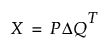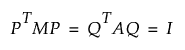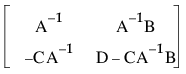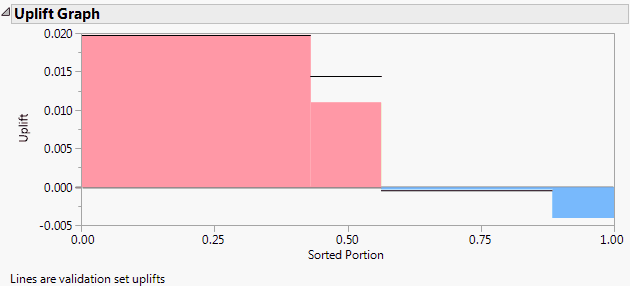Publication date: 08/13/2020

##Uplift Report Options

With the exception of the options described below, all of the red triangle options for the Uplift report are described in the documentation for the Partition platform. For more information about these options, see Partition Models in Predictive and Specialized Modeling.

###Minimum Size Split

This option presents a window where you enter a number or a fractional portion of the total sample size to define the minimum size split allowed. To specify a number, enter a value greater than or equal to 1. To specify a fraction of the sample size, enter a value less than 1. The default value for the Uplift platform is set to 25 or the floor of the number of rows divided by 2,000, whichever value is greater.

###Column Uplift Contributions

This table and plot address a column’s contribution to the uplift tree structure. A column’s contribution is computed as the sum of the F Ratio values associated with its splits. Recall that these values measure the significance of the treatment-by-split interaction term in the regression model.

###Uplift Graph

Consider the observations in the training set. Define uplift for an observation as the difference between the predicted probabilities or means across the levels of Treatment for the observation’s terminal node. These uplift values are sorted in descending order. On its vertical axis, the Uplift Graph shows the uplift values. On its horizontal axis, the graph shows the proportion of observations with each uplift value.

See Figure 6.5 for an example of an Uplift Graph for the Hair Care Product.jmp sample data table after three splits. Note that, for two groups of subjects (males and non-blond women in the Age 42 group), the promotion has a negative effect.

The horizontal lines shown on the Uplift Graph delineate the graph for the validation set. Specifically, the decision tree is evaluated for the validation set and the Uplift Graph is constructed from the estimated uplifts.

Figure 6.5 Uplift Graph###Save Columns

Save Difference

Saves the estimated difference in mean responses across levels of Treatment for the observation’s node. This is the estimated uplift.

Save Difference Formula

Saves the formula for the Difference, or uplift.

Publish Difference Formula

Creates the difference formula and saves it as a formula column script in the Formula Depot platform. If a Formula Depot report is not open, this option creates a Formula Depot report. See Formula Depot in Predictive and Specialized Modeling.